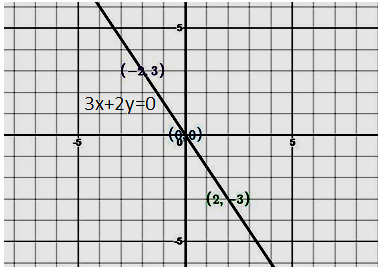Plot the line: $3x + 2y = 0$Verified
120.6k+ views
Hint: We have asked to plot a line for $3x + 2y = 0$. This is the linear equation in two variables and every linear equation in two variables is the equation of line. So to plot this firstly we have to find the point which satisfies this equation these are infinitely many points which will satisfy this equation. We will find only two points. These points will be enough to plot the line.
We first put $x = 0$in the equation, this will give the value of $y$. Again we put $y = 0$ in this equation this will give the value of $x$, and we get two points which help to plot the graph.

We have given equation
$3x + 2y = 0$ ------(i)
This is the equation of the line.
Putting $x = 0$in the equation (i)
$3.x.0 + 2y = 0$
$\Rightarrow$ $2y = 0$
$\Rightarrow$ $y = 0$
So for $x = 0$the value of $y = 0$ $(0,0)$ is the point on the line
Putting $x = 2$in the equation (i)
We get
$3.x.2 + 2y = 0$
$\Rightarrow$ $6 + 2y = 0$
$\Rightarrow$ $2y = - 6$
$\Rightarrow$ $y = - 3$
For $x = 2,y = - 3$
$(2,3)$ is the point on the line
Putting $y = - 3$ in the equation (i)
We get
$3x + 2 \times 3 = 0$
$\Rightarrow$ $3x + 6 = 0$
$\Rightarrow$ $3x = - 6$
$x = \dfrac{{ - 3}}{3} = - 2$
For $y = - 3$, $x = 2$
So $( - 2,3)$ is the point on the line

 $x$ $0$ $2$ $- 2$ $y$ $0$ $- 3$ $3$

Plotting these points in the graph and joining we get a straight line.Note: An equation is said to be a linear equation in two variables, if it is written in the form of $ax + by + c = 0$. Where $a, b$ and $c$ are real numbers and the coefficient of $x$ and $y$.
The solution of such equations is a pair of values, one for $x$ and one for $y$ which further moles the two sides of an equation equal.